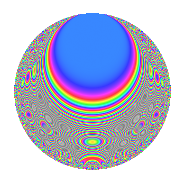# Properties

 Label 210.2.xLevel 210 Weight 2 Character orbit x Rep. character $$\chi_{210}(23,\cdot)$$ Character field $$\Q(\zeta_{12})$$ Dimension 64 Newform subspaces 1 Sturm bound 96 Trace bound 0

# Related objects

## Defining parameters

 Level: $$N$$ = $$210 = 2 \cdot 3 \cdot 5 \cdot 7$$ Weight: $$k$$ = $$2$$ Character orbit: $$[\chi]$$ = 210.x (of order $$12$$ and degree $$4$$) Character conductor: $$\operatorname{cond}(\chi)$$ = $$105$$ Character field: $$\Q(\zeta_{12})$$ Newform subspaces: $$1$$ Sturm bound: $$96$$ Trace bound: $$0$$

## Dimensions

The following table gives the dimensions of various subspaces of $$M_{2}(210, [\chi])$$.

Total New Old
Modular forms 224 64 160
Cusp forms 160 64 96
Eisenstein series 64 0 64

## Trace form

 $$64q + 8q^{6} + 4q^{7} + O(q^{10})$$ $$64q + 8q^{6} + 4q^{7} + 4q^{10} - 24q^{15} + 32q^{16} - 8q^{18} + 12q^{21} - 24q^{22} - 8q^{25} - 72q^{27} + 4q^{28} - 12q^{30} + 32q^{31} - 20q^{33} - 24q^{36} - 8q^{37} - 40q^{42} - 64q^{43} - 28q^{45} + 24q^{46} - 16q^{55} + 24q^{57} - 28q^{58} + 8q^{60} + 24q^{61} - 88q^{63} - 16q^{67} - 12q^{70} + 8q^{72} - 56q^{73} + 8q^{75} - 32q^{76} - 16q^{78} + 4q^{81} + 16q^{82} + 32q^{85} + 76q^{87} + 12q^{88} + 40q^{90} - 48q^{91} + 84q^{93} + 4q^{96} + 104q^{97} + O(q^{100})$$

## Decomposition of $$S_{2}^{\mathrm{new}}(210, [\chi])$$ into newform subspaces

Label Dim. $$A$$ Field CM Traces $q$-expansion
$$a_2$$ $$a_3$$ $$a_5$$ $$a_7$$
210.2.x.a $$64$$ $$1.677$$ None $$0$$ $$0$$ $$0$$ $$4$$

## Decomposition of $$S_{2}^{\mathrm{old}}(210, [\chi])$$ into lower level spaces

$$S_{2}^{\mathrm{old}}(210, [\chi]) \cong$$ $$S_{2}^{\mathrm{new}}(105, [\chi])$$$$^{\oplus 2}$$

## Hecke Characteristic Polynomials

There are no characteristic polynomials of Hecke operators in the database